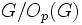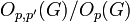# 3-step group for a prime

The article defines a property of groups, where the definition may be in terms of a particular prime that serves as parameter
View other prime-parametrized group properties | View other group properties

## Definition

Suppose$G$ is a finite group and$p$ is a prime number. We say that$G$ is a 3-step group with respect to$p$:

1.$O_{p,p'}(G)$ is a Frobenius group with Frobenius kernel$O_p(G)$ and cyclic complement of odd order. In particular, this means that either$p = 2$ or$G$ has odd order.
2.$G = O_{p,p',p}(G)$ and strictly contains$O_{p,p'}(G)$.
3.$G/O_p(G)$ is a Frobenius group with Frobenius kernel$O_{p,p'}(G)/O_p(G)$.

## Examples

Symmetric group:S4 is an example of a 3-step group with$p = 2$. If$G = S_4$, then:

•$O_p(G)$ is the normal Klein four-subgroup of symmetric group:S4, isomorphic to the Klein four-group.
•$O_{p,p'}(G)$ is A4 in S4, isomorphic to alternating group:A4. This is a Frobenius group with$O_p(G)$ as its Frobenius kernel and complement cyclic group:Z3.
•$G/O_p(G)$ is isomorphic to symmetric group:S3, and has Frobenius kernel A3 in S3, which is the image mod$O_p(G)$ of A4 in S4, which is$O_{p,p'}(G)$.